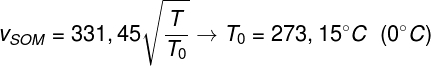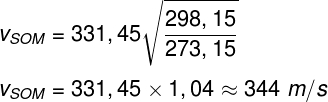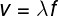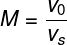Mechanics

# Speed ​​of sound

Velocity of sound is a measure of the space that a sound wave travels over a period of time. This measurement depends directly on the medium in which the sound propagates. Furthermore, the speed of sound depends on a characteristic of the medium in which it propagates called elasticity , that is, its ability to deform when subjected to some pressure.

For a better understanding of all the content contained in this text, we suggest you check out the articles What is sound? and also Sound waves .

## Characteristics

When sound travels through the air, for example, it creates regions of compression and rarefaction. Such regions are characterized by high and low pressures that alternate in a back-and-forth motion. It is for this reason that sound is defined as a transverse and mechanical wave : it propagates in the same direction as the stimulus responsible for producing it. Also, there needs to be a way to propagate it.

In addition to its dependence on the stiffness of the medium, the speed of sound can also vary with temperature. In the case of sound propagating in atmospheric air, there is an empirical formula that relates the speed of sound propagation from 0 °C (273.15 K) to the temperature of the air. Check out:The formula above relates the speed of sound propagation at 0ºC (331.45 m/s) with the temperature at which you want to calculate the speed of sound (T), in kelvin. Check out the following example, in which we calculated the speed of sound in air for a temperature of 25 °C (298.15 K):The result obtained by the above calculation is approximate, since different factors, such as height above sea level, can directly affect the propagation speed of sound in the air. If converted to kilometers per hour (km/h), the speed of sound in air obtained from this calculation is approximately 1238 km/h .

Although useful, the formula shown above is only valid for sound propagation in atmospheric air. If we want to use a more general formula, it is necessary to consider the formula that relates the speed of sound to its frequency and wavelength.

See also: Check out how much physics there is behind musical instruments

## Formulas for calculating the speed of sound

It is possible to calculate the speed of sound if we know its wavelength and frequency . We call the wavelength the space that is traversed by a complete oscillation of the sound wave, while the frequency can be understood as the number of oscillations that the sound wave makes every second:v – speed of sound (m/s)

λ – wavelength (m)

f – sound frequency (s -1 or Hz)

Another interesting fact about sound speed is that its propagation speed does not depend on the observer (who listens to the sound waves). If you move away from or approach a source emitting sound waves, your speed relative to those waves remains constant.

This happens because the speed of propagation of sound depends only on the characteristics of the medium in which it propagates. Thus, when we approach a source emitting sound waves, we perceive an increase in the frequency of the sound, accompanied by a decrease in the wavelength, and the propagation speed remains constant. The name given to this phenomenon is the doppler effect. If you want to know more about how this phenomenon happens, access our text: Doppler effect .

When sound passes from one medium to another, it can be refracted , just as light does. When refracted, sound can either have its speed increased or decreased, according to the rigidity of the medium in which it starts to propagate. When sound is transmitted through the interior of solid media, as in metals, its propagation speeds are generally much higher than those achieved in liquid and gaseous media, thanks to the close proximity and strong interaction between the molecules of a solid.

In the interior of solids, unlike the way in which sound propagates in air, sounds can propagate in the form of transverse waves , as well as in the form of longitudinal waves. The longitudinal waves produced by sound inside solids are called pressure waves This type of wave can be observed and detected over long distances during the occurrence of large earthquakes , for example.

## Speed ​​of sound in Mach

There is a number used as a parameter to compare the speed of propagation of something with respect to the average speed of propagation of sound in the air (340 m/s). This number is called the Mach number (Ma) and is widely used to compare the flight speed of fighter jets and rockets, which often travel at speeds greater than the speed of sound, called supersonic speeds Here’s the formula used to calculate the Mach number:M – Mach number

vO  object speed

S – average speed of sound in air (340 m/s)

If we analyze the formula used to calculate the Mach number, we will realize that it is a dimensionless quantity (deprived of any physical unit that defines it). Furthermore, when some body moves at 2 Ma (read 2 Mach), it means that its propagation speed is 2 times greater than the propagation speed of sound in air (680 m/s).

## Speed ​​of sound on strings

When sound propagates in some one-dimensional and homogeneous medium, such as strings or stretched wires, it is possible to determine its speed by means of Brook Taylor ‘s formula . Check out:v – sound propagation speed (m/s)

F – Traction applied to the wire (N)

μ – linear density of the yarn (kg/m)

The formula above shows that the speed of sound propagation in strings and wires is proportional to the intensity of the traction force applied to them: the more stretched, the greater the speed of sound inside them. Furthermore, it can be seen that the speed of sound is inversely proportional to the quantity μ , known as linear density. This quantity measures the amount of mass, in kilograms, contained in each meter of length of this wire.

## Speed ​​of sound in different media

Check the speed of sound for different media:

 Quite Speed ​​of sound (m/s) Aluminum 6420 Steel 5960 Iron 5950 Distilled water 1498 Sea water 1531 Hydrogen 1284 Helium 965 Oxygen 316

When we analyze the table above, it is possible to notice that there is a big difference between the speed of sound in pure water (distilled) and in sea water (salt). This is due to the presence of a large amount of diluted salts in seawater, which are very effective in propagating sound waves.